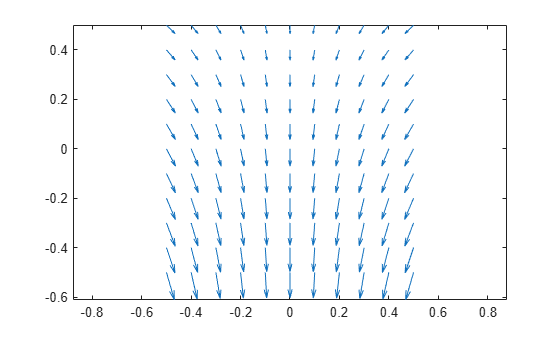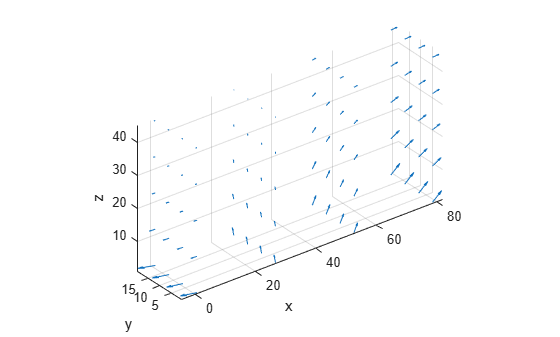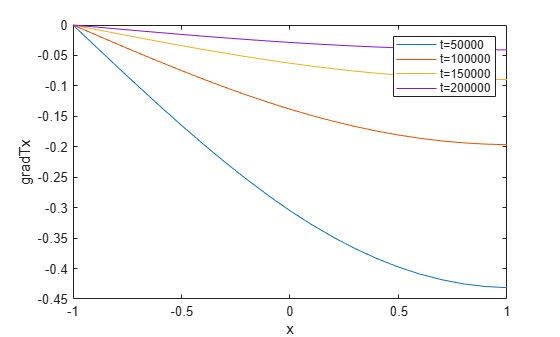Evaluate temperature gradient of a thermal solution at arbitrary spatial locations

## Syntax

``````[gradTx,gradTy] = evaluateTemperatureGradient(thermalresults,xq,yq)``````
``````[gradTx,gradTy,gradTz] = evaluateTemperatureGradient(thermalresults,xq,yq,zq)``````
``[___] = evaluateTemperatureGradient(thermalresults,querypoints)``
``[___] = evaluateTemperatureGradient(___,iT)``

## Description

example

``````[gradTx,gradTy] = evaluateTemperatureGradient(thermalresults,xq,yq)``` returns the interpolated values of temperature gradients of the thermal model solution `thermalresults` at the 2-D points specified in `xq` and `yq`. This syntax is valid for both the steady-state and transient thermal models.```

example

``````[gradTx,gradTy,gradTz] = evaluateTemperatureGradient(thermalresults,xq,yq,zq)``` returns the interpolated temperature gradients at the 3-D points specified in `xq`, `yq`, and `zq`. This syntax is valid for both the steady-state and transient thermal models.```

example

````[___] = evaluateTemperatureGradient(thermalresults,querypoints)` returns the interpolated values of the temperature gradients at the points specified in `querypoints`. This syntax is valid for both the steady-state and transient thermal models.```

example

````[___] = evaluateTemperatureGradient(___,iT)` returns the interpolated values of the temperature gradients for the time-dependent equation at times `iT`. Specify `iT` after the input arguments in any of the previous syntaxes.The first dimension of `gradTx`, `gradTy`, and, in 3-D case, `gradTz` corresponds to query points. The second dimension corresponds to time-steps `iT`.```

## Examples

collapse all

For a 2-D steady-state thermal model, evaluate temperature gradients at the nodal locations and at the points specified by `x` and `y` coordinates.

Create a thermal model for steady-state analysis.

`thermalmodel = createpde("thermal");`

Create the geometry and include it in the model.

```R1 = [3,4,-1,1,1,-1,1,1,-1,-1]'; g = decsg(R1,'R1',('R1')'); geometryFromEdges(thermalmodel,g); pdegplot(thermalmodel,"EdgeLabels","on") xlim([-1.5 1.5]) axis equal```Assuming that this geometry represents an iron plate, the thermal conductivity is $79.5\phantom{\rule{0.16666666666666666em}{0ex}}W/\left(mK\right)$.

`thermalProperties(thermalmodel,"ThermalConductivity",79.5,"Face",1);`

Apply a constant temperature of 300 K to the bottom of the plate (edge 3). Also, assume that the top of the plate (edge 1) is insulated, and apply convection on the two sides of the plate (edges 2 and 4).

```thermalBC(thermalmodel,"Edge",3,"Temperature",300); thermalBC(thermalmodel,"Edge",1,"HeatFlux",0); thermalBC(thermalmodel,"Edge",[2 4], ... "ConvectionCoefficient",25, ... "AmbientTemperature",50);```

Mesh the geometry and solve the problem.

```generateMesh(thermalmodel); results = solve(thermalmodel)```
```results = SteadyStateThermalResults with properties: Temperature: [1541x1 double] XGradients: [1541x1 double] YGradients: [1541x1 double] ZGradients: [] Mesh: [1x1 FEMesh] ```

The solver finds the temperatures and temperature gradients at the nodal locations. To access these values, use `results.Temperature`, `results.XGradients`, and so on. For example, plot the temperature gradients at nodal locations.

```figure; pdeplot(thermalmodel, ... "FlowData",[results.XGradients results.YGradients]);```Create a grid specified by `x` and `y` coordinates, and evaluate temperature gradients to the grid.

```v = linspace(-0.5,0.5,11); [X,Y] = meshgrid(v); [gradTx,gradTy] = ... evaluateTemperatureGradient(results,X,Y);```

Reshape the `gradTx` and `gradTy` vectors, and plot the resulting temperature gradients.

```gradTx = reshape(gradTx,size(X)); gradTy = reshape(gradTy,size(Y)); figure quiver(X,Y,gradTx,gradTy)```Alternatively, you can specify the grid by using a matrix of query points.

```querypoints = [X(:) Y(:)]'; [gradTx,gradTy] = ... evaluateTemperatureGradient(results,querypoints); gradTx = reshape(gradTx,size(X)); gradTy = reshape(gradTy,size(Y)); figure quiver(X,Y,gradTx,gradTy)```For a 3-D steady-state thermal model, evaluate temperature gradients at the nodal locations and at the points specified by `x`, `y`, and `z` coordinates.

Create a thermal model for steady-state analysis.

`thermalmodel = createpde("thermal");`

Create the following 3-D geometry and include it in the model.

```importGeometry(thermalmodel,"Block.stl"); pdegplot(thermalmodel,"FaceLabels","on","FaceAlpha",0.5) title("Copper block, cm") axis equal```Assuming that this is a copper block, the thermal conductivity of the block is approximately $4\phantom{\rule{0.16666666666666666em}{0ex}}W/\left(cmK\right)$.

`thermalProperties(thermalmodel,"ThermalConductivity",4);`

Apply a constant temperature of 373 K to the left side of the block (edge 1) and a constant temperature of 573 K to the right side of the block.

```thermalBC(thermalmodel,"Face",1,"Temperature",373); thermalBC(thermalmodel,"Face",3,"Temperature",573);```

Apply a heat flux boundary condition to the bottom of the block.

`thermalBC(thermalmodel,"Face",4,"HeatFlux",-20);`

Mesh the geometry and solve the problem.

```generateMesh(thermalmodel); thermalresults = solve(thermalmodel)```
```thermalresults = SteadyStateThermalResults with properties: Temperature: [12691x1 double] XGradients: [12691x1 double] YGradients: [12691x1 double] ZGradients: [12691x1 double] Mesh: [1x1 FEMesh] ```

The solver finds the values of temperatures and temperature gradients at the nodal locations. To access these values, use `results.Temperature`, `results.XGradients`, and so on.

Create a grid specified by `x`, `y`, and `z` coordinates, and evaluate temperature gradients to the grid.

```[X,Y,Z] = meshgrid(1:26:100,1:6:20,1:11:50); [gradTx,gradTy,gradTz] = ... evaluateTemperatureGradient(thermalresults,X,Y,Z);```

Reshape the `gradTx`, `gradTy`, and `gradTz` vectors, and plot the resulting temperature gradients.

```gradTx = reshape(gradTx,size(X)); gradTy = reshape(gradTy,size(Y)); gradTz = reshape(gradTz,size(Z)); figure quiver3(X,Y,Z,gradTx,gradTy,gradTz) axis equal xlabel("x") ylabel("y") zlabel("z")```Alternatively, you can specify the grid by using a matrix of query points.

```querypoints = [X(:) Y(:) Z(:)]'; [gradTx,gradTy,gradTz] = ... evaluateTemperatureGradient(thermalresults,querypoints); gradTx = reshape(gradTx,size(X)); gradTy = reshape(gradTy,size(Y)); gradTz = reshape(gradTz,size(Z)); figure quiver3(X,Y,Z,gradTx,gradTy,gradTz) axis equal xlabel("x") ylabel("y") zlabel("z")```Solve a 2-D transient heat transfer problem on a square domain and compute temperature gradients at the convective boundary.

Create a transient thermal model for this problem.

`thermalmodel = createpde("thermal","transient");`

Create the geometry and include it in the model.

```g = @squareg; geometryFromEdges(thermalmodel,g); pdegplot(thermalmodel,"EdgeLabels","on") xlim([-1.2 1.2]) ylim([-1.2 1.2]) axis equal```Assign the following thermal properties: thermal conductivity is $100\phantom{\rule{0.16666666666666666em}{0ex}}W/\left({m}^{\circ }C\right)$, mass density is $7800\phantom{\rule{0.16666666666666666em}{0ex}}kg/{m}^{3}$, and specific heat is $500\phantom{\rule{0.16666666666666666em}{0ex}}J/\left(k{g}^{\circ }C\right)$.

```thermalProperties(thermalmodel,"ThermalConductivity",100, ... "MassDensity",7800, ... "SpecificHeat",500);```

Apply insulated boundary conditions on three edges and the free convection boundary condition on the right edge.

```thermalBC(thermalmodel,"Edge",[1 3 4],"HeatFlux",0); thermalBC(thermalmodel,"Edge",2, ... "ConvectionCoefficient",5000, ... "AmbientTemperature",25);```

Set the initial conditions: uniform room temperature across domain and higher temperature on the left edge.

```thermalIC(thermalmodel,25); thermalIC(thermalmodel,100,"Edge",4);```

Generate a mesh and solve the problem using `0:1000:200000` as a vector of times.

```generateMesh(thermalmodel); tlist = 0:1000:200000; thermalresults = solve(thermalmodel,tlist);```

Define a line at convection boundary and compute temperature gradients across that line.

```X = -1:0.1:1; Y = ones(size(X)); [gradTx,gradTy] = evaluateTemperatureGradient(thermalresults, ... X,Y,1:length(tlist));```

Plot the interpolated gradient component `gradTx` along the `x` axis for the following values from the time interval `tlist`.

```figure t = [51:50:201]; for i = t p(i) = plot(X,gradTx(:,i),"DisplayName", ... strcat("t=",num2str(tlist(i)))); hold on end legend(p(t)) xlabel("x") ylabel("gradTx")```## Input Arguments

collapse all

Solution of a thermal problem, specified as a `SteadyStateThermalResults` object or a `TransientThermalResults` object. Create `thermalresults` using the `solve` function.

Example: ```thermalresults = solve(thermalmodel)```

x-coordinate query points, specified as a real array. `evaluateTemperatureGradient` evaluates temperature gradient at the 2-D coordinate points `[xq(i) yq(i)]` or at the 3-D coordinate points `[xq(i) yq(i) zq(i)]`. So `xq`, `yq`, and (if present) `zq` must have the same number of entries.

`evaluateTemperatureGradient` converts query points to column vectors `xq(:)`, `yq(:)`, and (if present) `zq(:)`. It returns the temperature gradient in a form of a column vector of the same size. To ensure that the dimensions of the returned solution is consistent with the dimensions of the original query points, use `reshape`. For example, use `gradTx = reshape(gradTx,size(xq))`.

Data Types: `double`

y-coordinate query points, specified as a real array. `evaluateTemperatureGradient` evaluates the temperature gradient at the 2-D coordinate points ```[xq(i) yq(i)]``` or at the 3-D coordinate points ```[xq(i) yq(i) zq(i)]```. So `xq`, `yq`, and (if present) `zq` must have the same number of entries.

`evaluateTemperatureGradient` converts query points to column vectors `xq(:)`, `yq(:)`, and (if present) `zq(:)`. It returns the temperature gradient in a form of a column vector of the same size. To ensure that the dimensions of the returned solution is consistent with the dimensions of the original query points, use `reshape`. For example, use `gradTy = reshape(gradTy,size(yq))`.

Data Types: `double`

z-coordinate query points, specified as a real array. `evaluateTemperatureGradient` evaluates the temperature gradient at the 3-D coordinate points ```[xq(i) yq(i) zq(i)]```. So `xq`, `yq`, and `zq` must have the same number of entries.

`evaluateTemperatureGradient` converts query points to column vectors `xq(:)`, `yq(:)`, and (if present) `zq(:)`. It returns the temperature gradient in a form of a column vector of the same size. To ensure that the dimensions of the returned solution is consistent with the dimensions of the original query points, use `reshape`. For example, use `gradTz = reshape(gradTz,size(zq))`.

Data Types: `double`

Query points, specified as a real matrix with either two rows for 2-D geometry, or three rows for 3-D geometry. `evaluateTemperatureGradient` evaluates the temperature gradient at the coordinate points `querypoints(:,i)`, so each column of `querypoints` contains exactly one 2-D or 3-D query point.

Example: For 2-D geometry, ```querypoints = [0.5 0.5 0.75 0.75; 1 2 0 0.5]```

Data Types: `double`

Time indices, specified as a vector of positive integers. Each entry in `iT` specifies a time index.

Example: `iT = 1:5:21` specifies every fifth time-step up to 21.

Data Types: `double`

## Output Arguments

collapse all

x-component of the temperature gradient, returned as a matrix. For query points that are outside the geometry, `gradTx` = `NaN`.

y-component of the temperature gradient, returned as a matrix. For query points that are outside the geometry, `gradTy` = `NaN`.

z-component of the temperature gradient, returned as a matrix. For query points that are outside the geometry, `gradTz` = `NaN`.

## Version History

Introduced in R2017a